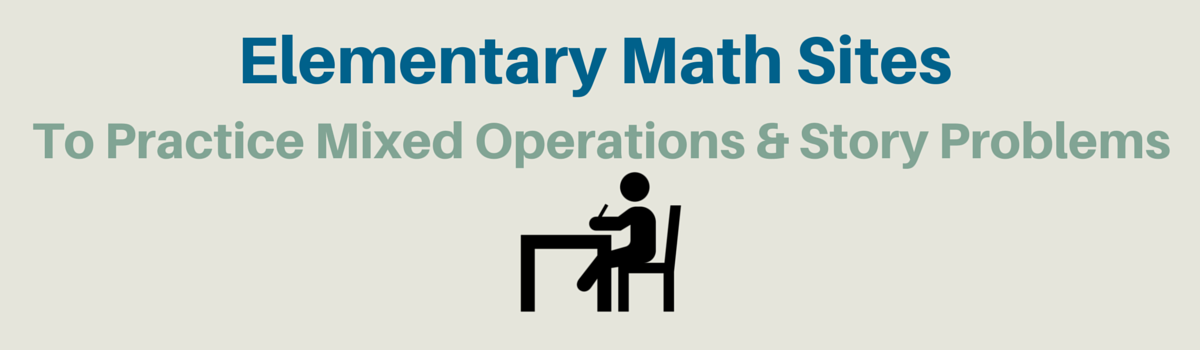Updated by Jon Samuelson on Nov 04, 2019REPORTJon Samuelson
Owner
19 items   18 followers   0 votes   5.4k views

# Elementary Math Websites To Practice Mixed Operations/Story Problems

A list developed by Cindy Lawrence, 3rd grade teacher in Texas. This list accompanies Unit 8 of the Third Grade Everyday Math Curriculum.

1

## Yummy Math | We provide teachers and students with mathematics relevant to our world today ...Professional athletes make a lot of money. Aaron Rodgers recently signed a five year, 110 million dollar contract with Green Bay Packers! Did you know that professional athletes have to pay an amazing amount of federal, state, and sometimes even city tax?

2

## Becoming Lord Voldemath - A Math Wizard Game "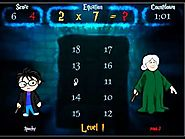The object of the game is to defeat four wizards in a game of mathematics by answering math problems correctly AND before your wizard opponent does. If you succeed, you will morph into the all powerful mathematician - Lord Voldemath! First choose the operation and then the numbers (tables) to include in the problems. Then, name the wizards!

3

## Math Word Problems - Math HoopsAction packed math site for elementary and middle school students featuring math games, math word problems, math worksheets, logic puzzles, and math videos.

4

## Math Word Problems - Grand Slam Math +/-Action packed math site for elementary and middle school students featuring math games, math word problems, math worksheets, logic puzzles, and math videos.

5

## Math Word Problems - Grand Slam Math x/Action packed math site for elementary and middle school students featuring math games, math word problems, math worksheets, logic puzzles, and math videos.

6

## Math Word Problems - Problems With Katie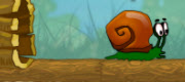Action packed math site for elementary and middle school students featuring math games, math word problems, math worksheets, logic puzzles, and math videos.

7

## Math Word Problems - Word Problem Database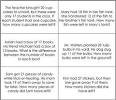Action packed math site for elementary and middle school students featuring math games, math word problems, math worksheets, logic puzzles, and math videos.

8

## Solve It Math VideosAction packed math site for elementary and middle school students featuring math games, math word problems, math worksheets, logic puzzles, and math videos.

9

## BBC - Problem solving activity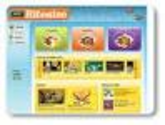An animated KS2 activity for the Maths topic: Problem solving

10

## ABCya! Math Match | Kids Practice Addition, Subtraction, Multiplication & DivisionABCya! Math Match is a fun way for students to practice math facts. There are three activities to choose from: Addition, Subtraction, or Multiplication. The goal is to flip cards to match equations with answers or answers with equations. There is a fun bonus round between levels!

11

## ABCya! Math Quiz - Take a quiz and print your results!ABCya! Math Quiz is a great way to check your math skills! Children pick from four math quizzes: Addition, Subtraction, Multiplication & Division. Results are provided after each math quiz.

12

## Monkey Drive - Racing Math Games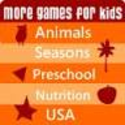Fun driving math game for kids. Drive the monkey car and practice math!

13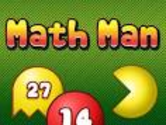Arcade style math game for kids. A fun way to learn math!

14

## Math Games: Quick Calculate Level 1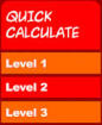Practice addition, multiplication, and subtraction with this free math game.

15

## Fruit Shoot Game: Compare EquationsLearn how to compare equations - using greater than, less than, equal to - with this fun shooting game.

16

## BBC - Cross the Swamp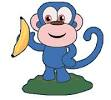Help guide the monkey across the swamp by answering math problems.

17

## Mr.N’s Math Slalom | MrNussbaum.com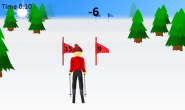Guide the skier through the slaloms by answering math problems correctly.

18

## Tackle Math Ball | MrNussbaum.com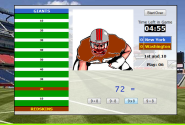Nothing like some tackle math ball to encourage students to practice their math.

19

## World Cup Math | MrNussbaum.com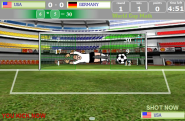Practice math with the world's most popular sport.